# LESSON 8 4 Trigonometry FiveMinute Check over Lesson

• Slides: 41LESSON 8– 4 TrigonometryFive-Minute Check (over Lesson 8– 3) TEKS Then/Now New Vocabulary Key Concept: Trigonometric Ratios Example 1: Find Sine, Cosine, and Tangent Ratios Example 2: Use Special Right Triangles to Find Trigonometric Ratios Example 3: Real-World Example: Estimate Measures Using Trigonometry Key Concept: Inverse Trigonometric Ratios Example 4: Find Angle Measures Using Inverse Trigonometric Ratios Example 5: Solve a Right Triangle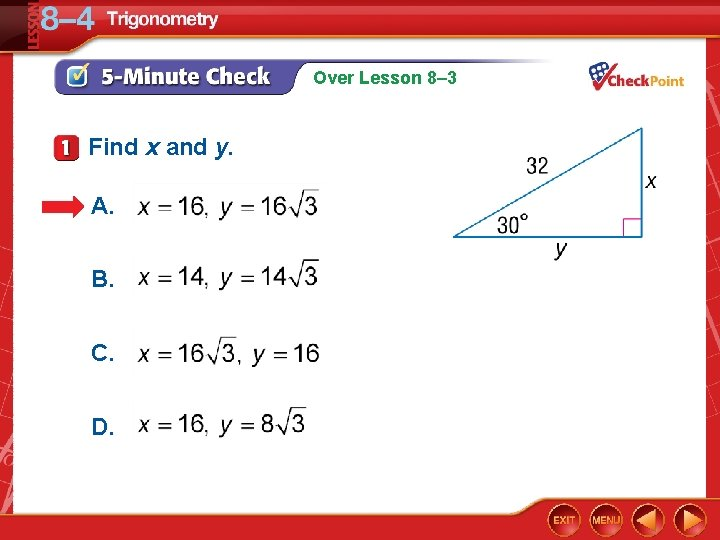Over Lesson 8– 3 Find x and y. A. B. C. D.Over Lesson 8– 3 Find x and y. A. x = 5, y = 5 B. x = 5, y = 45 C. D.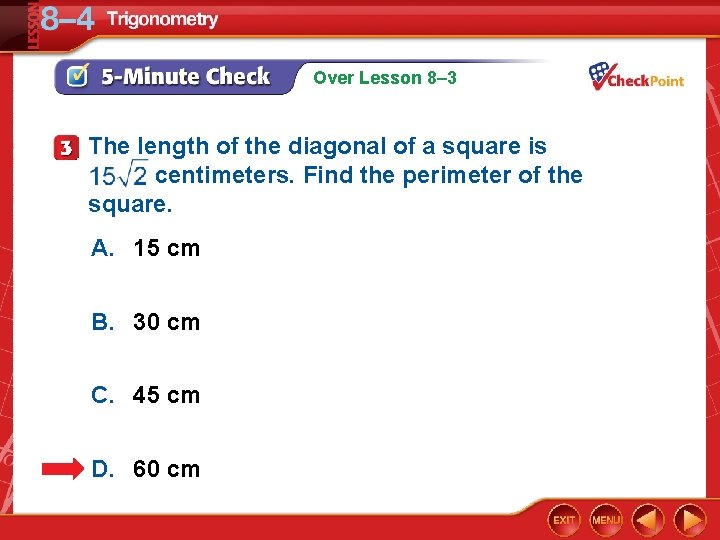Over Lesson 8– 3 The length of the diagonal of a square is centimeters. Find the perimeter of the square. A. 15 cm B. 30 cm C. 45 cm D. 60 cm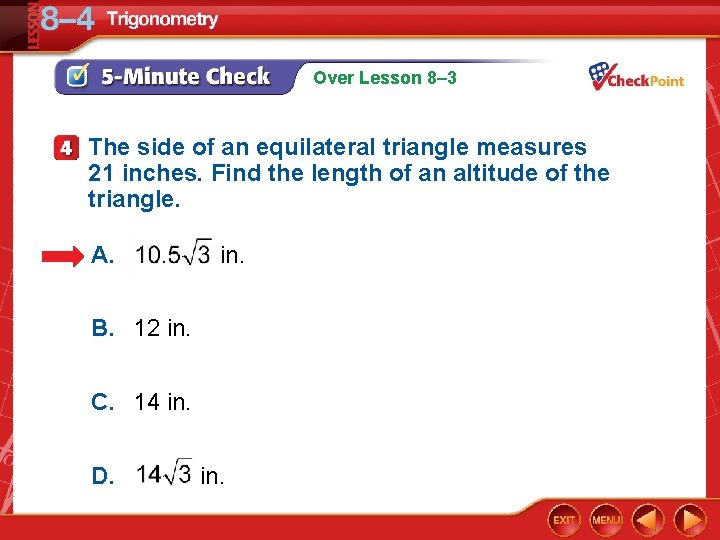Over Lesson 8– 3 The side of an equilateral triangle measures 21 inches. Find the length of an altitude of the triangle. A. in. B. 12 in. C. 14 in. D. in.Over Lesson 8– 3 ΔMNP is a 45°-90° triangle with right angle P. Find the coordinates of M in Quadrant II for P(2, 3) and N(2, 8). A. (– 1, 3) B. (– 3, 3) C. (5, 3) D. (6, 2)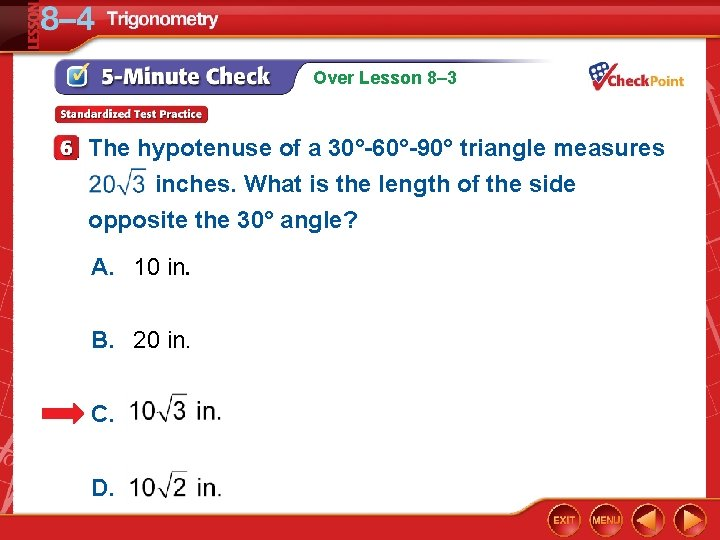Over Lesson 8– 3 The hypotenuse of a 30°-60°-90° triangle measures inches. What is the length of the side opposite the 30° angle? A. 10 in. B. 20 in. C. D.Targeted TEKS G. 9(A) Determine the lengths of sides and measures of angles in a right triangle by applying the trigonometric ratios sine, cosine, and tangent to solve problems. Mathematical Processes G. 1(C), G. 1(G)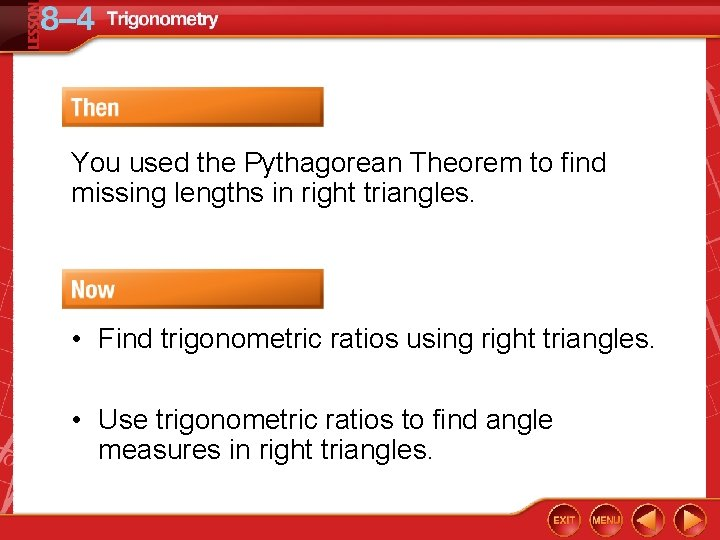You used the Pythagorean Theorem to find missing lengths in right triangles. • Find trigonometric ratios using right triangles. • Use trigonometric ratios to find angle measures in right triangles.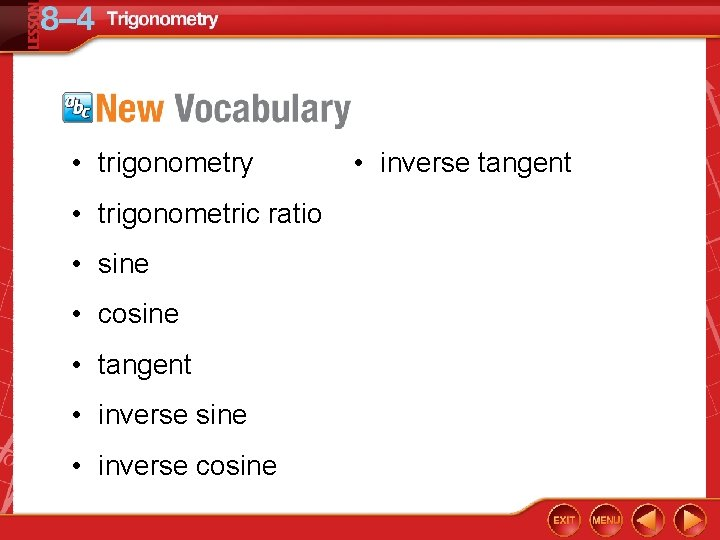• trigonometry • trigonometric ratio • sine • cosine • tangent • inverse sine • inverse cosine • inverse tangent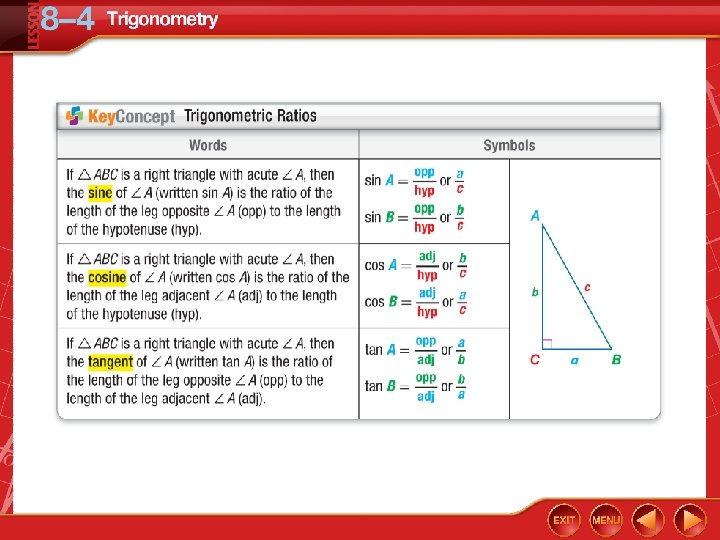Find Sine, Cosine, and Tangent Ratios A. Express sin L as a fraction and as a decimal to the nearest hundredth. Answer:Find Sine, Cosine, and Tangent Ratios B. Express cos L as a fraction and as a decimal to the nearest hundredth. Answer:Find Sine, Cosine, and Tangent Ratios C. Express tan L as a fraction and as a decimal to the nearest hundredth. Answer: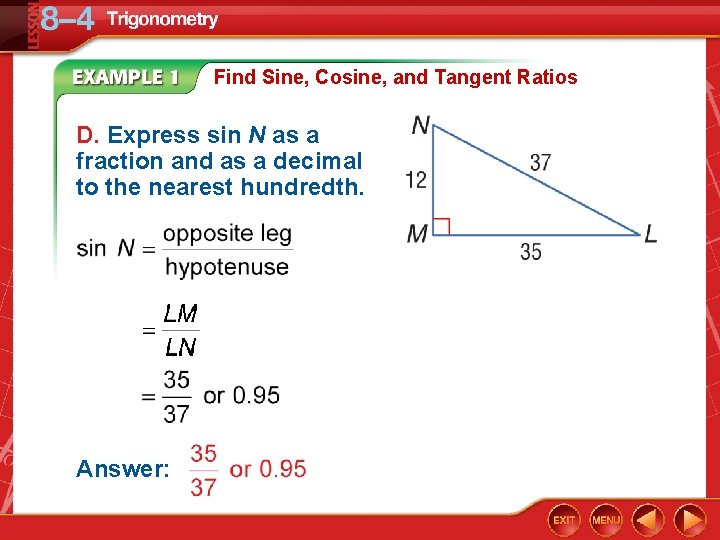Find Sine, Cosine, and Tangent Ratios D. Express sin N as a fraction and as a decimal to the nearest hundredth. Answer: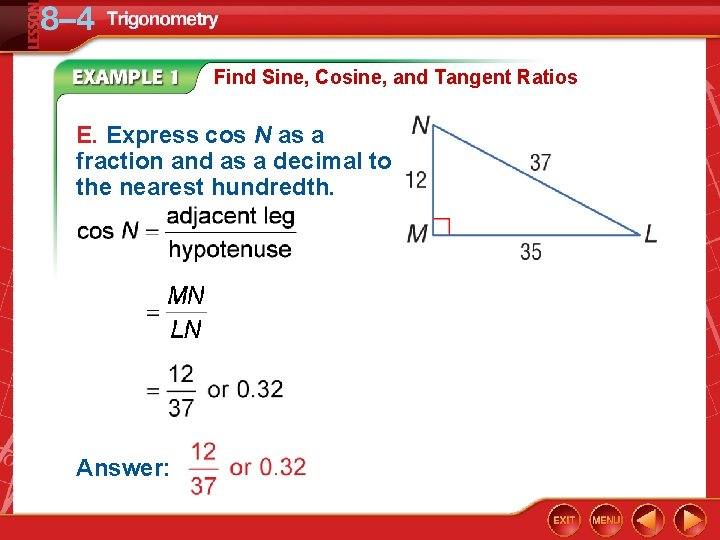Find Sine, Cosine, and Tangent Ratios E. Express cos N as a fraction and as a decimal to the nearest hundredth. Answer: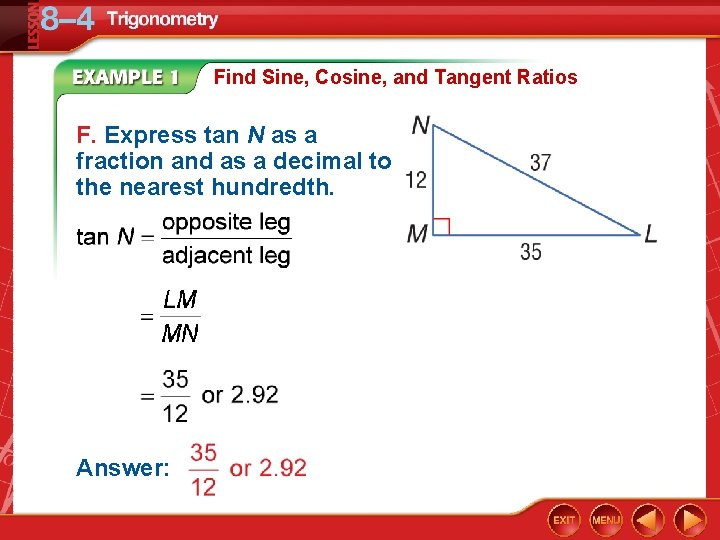Find Sine, Cosine, and Tangent Ratios F. Express tan N as a fraction and as a decimal to the nearest hundredth. Answer:A. Find sin A. A. B. C. D.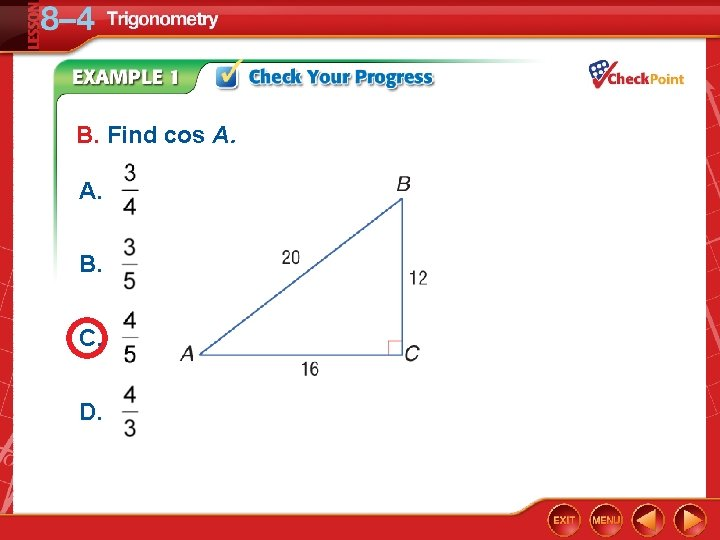B. Find cos A. A. B. C. D.C. Find tan A. A. B. C. D.D. Find sin B. A. B. C. D.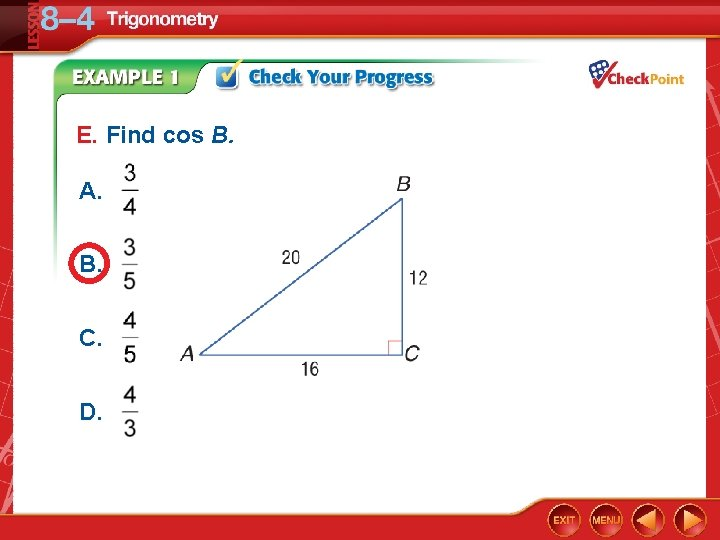E. Find cos B. A. B. C. D.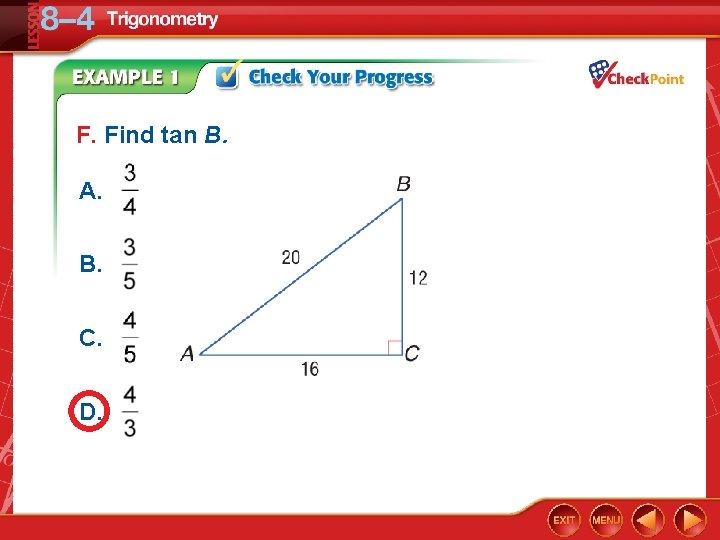F. Find tan B. A. B. C. D.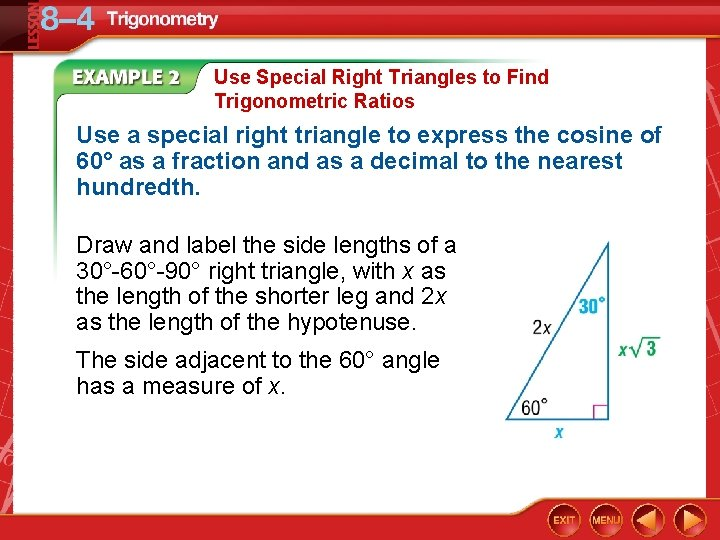Use Special Right Triangles to Find Trigonometric Ratios Use a special right triangle to express the cosine of 60° as a fraction and as a decimal to the nearest hundredth. Draw and label the side lengths of a 30°-60°-90° right triangle, with x as the length of the shorter leg and 2 x as the length of the hypotenuse. The side adjacent to the 60° angle has a measure of x.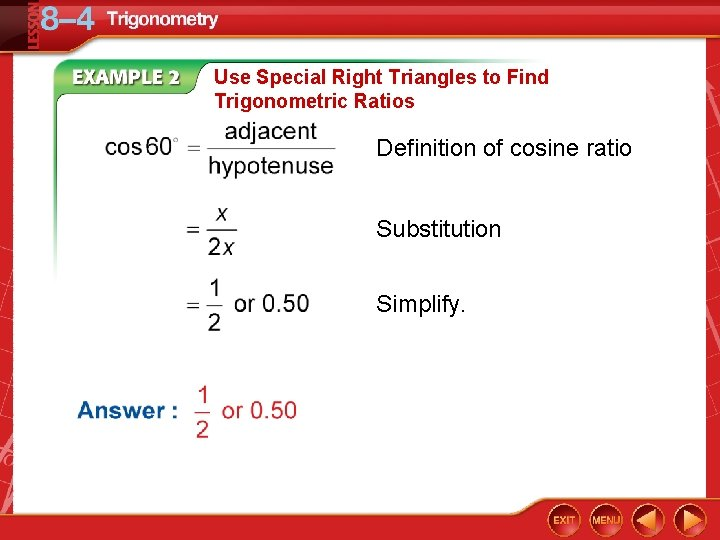Use Special Right Triangles to Find Trigonometric Ratios Definition of cosine ratio Substitution Simplify.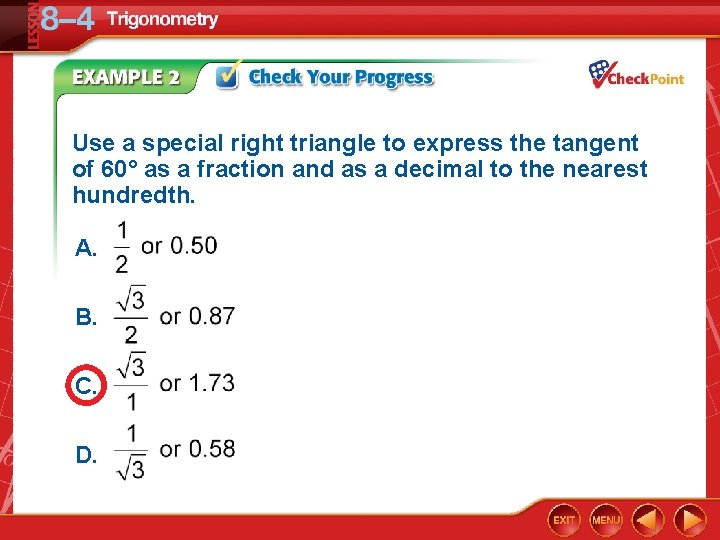Use a special right triangle to express the tangent of 60° as a fraction and as a decimal to the nearest hundredth. A. B. C. D.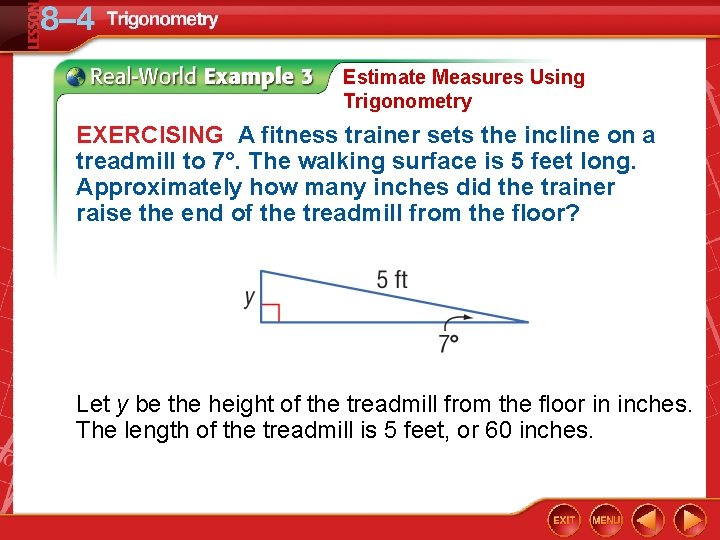Estimate Measures Using Trigonometry EXERCISING A fitness trainer sets the incline on a treadmill to 7°. The walking surface is 5 feet long. Approximately how many inches did the trainer raise the end of the treadmill from the floor? Let y be the height of the treadmill from the floor in inches. The length of the treadmill is 5 feet, or 60 inches.Estimate Measures Using Trigonometry Multiply each side by 60. Use a calculator to find y. KEYSTROKES: 60 SIN 7 ENTER 7. 312160604 Answer: The treadmill is about 7. 3 inches high.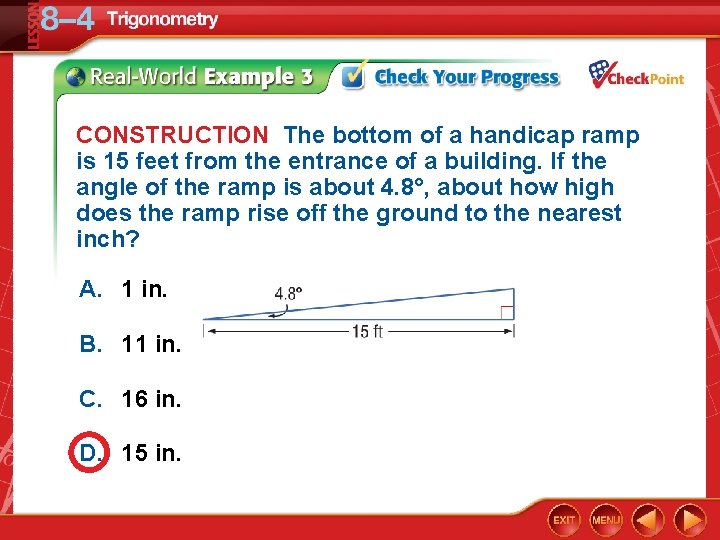CONSTRUCTION The bottom of a handicap ramp is 15 feet from the entrance of a building. If the angle of the ramp is about 4. 8°, about how high does the ramp rise off the ground to the nearest inch? A. 1 in. B. 11 in. C. 16 in. D. 15 in.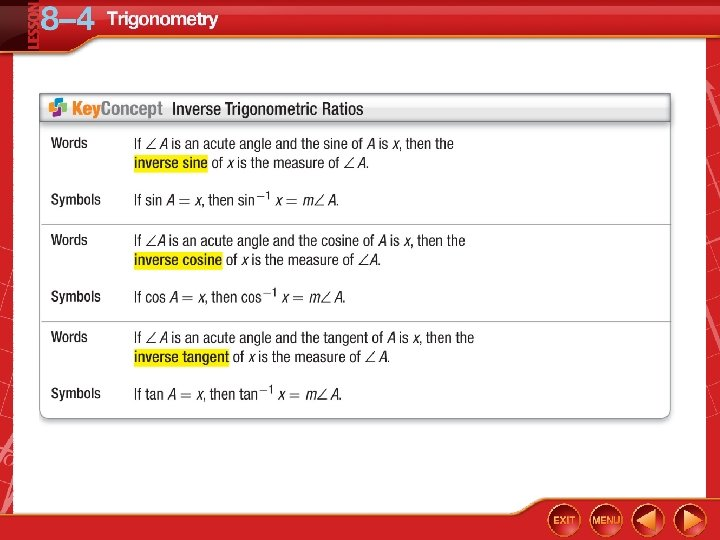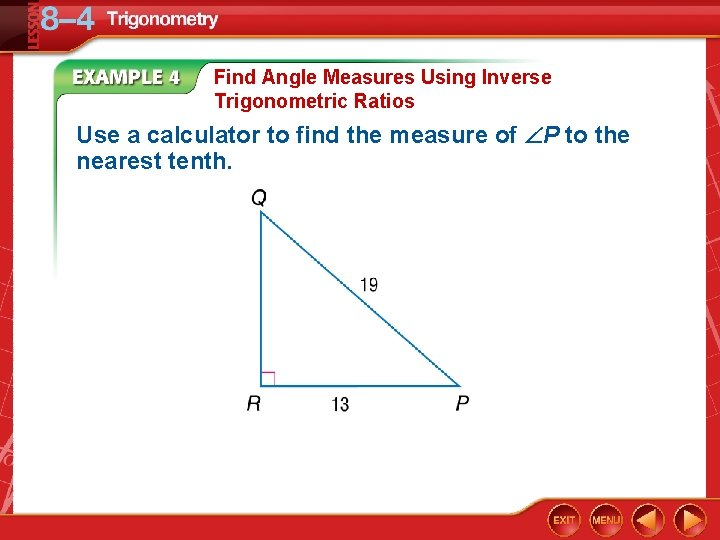Find Angle Measures Using Inverse Trigonometric Ratios Use a calculator to find the measure of P to the nearest tenth.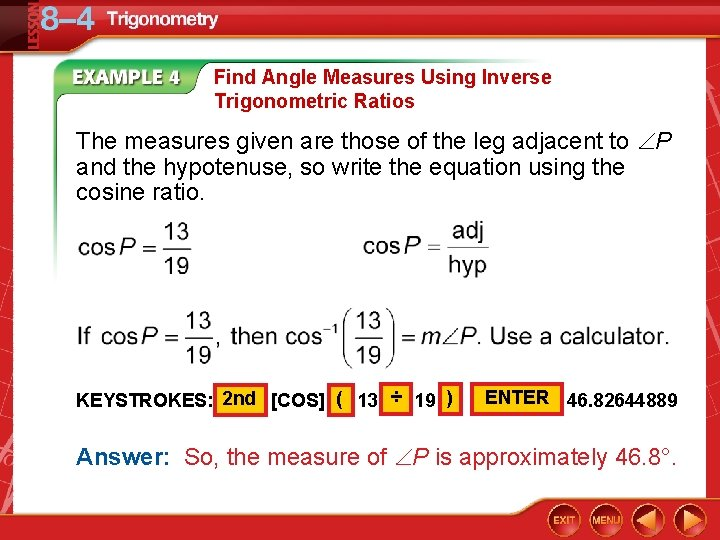Find Angle Measures Using Inverse Trigonometric Ratios The measures given are those of the leg adjacent to P and the hypotenuse, so write the equation using the cosine ratio. KEYSTROKES: 2 nd [COS] ( 13 ÷ 19 ) ENTER 46. 82644889 Answer: So, the measure of P is approximately 46. 8°.Use a calculator to find the measure of D to the nearest tenth. A. 44. 1° B. 48. 3° C. 55. 4° D. 57. 2°Solve a Right Triangle Solve the right triangle. Round side measures to the nearest hundredth and angle measures to the nearest degree.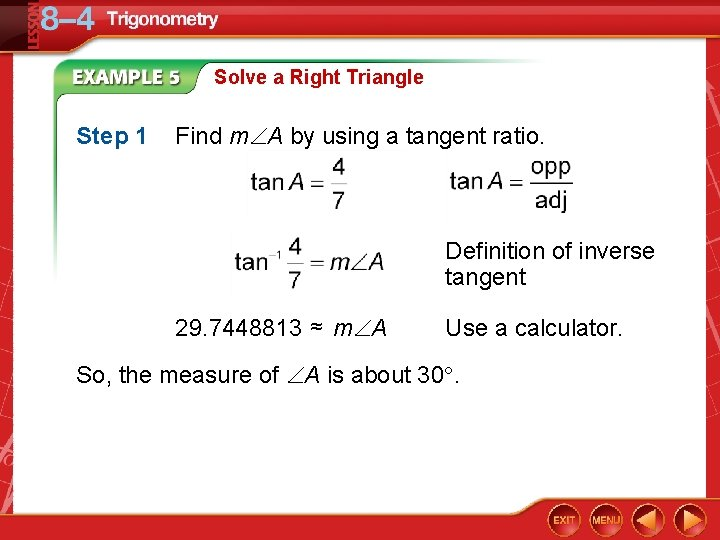Solve a Right Triangle Step 1 Find m A by using a tangent ratio. Definition of inverse tangent 29. 7448813 ≈ m A Use a calculator. So, the measure of A is about 30.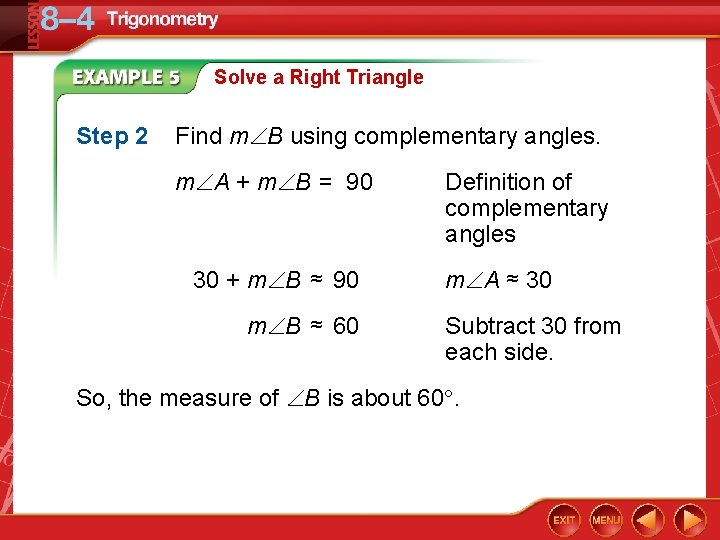Solve a Right Triangle Step 2 Find m B using complementary angles. m A + m B = 90 30 + m B ≈ 90 m B ≈ 60 Definition of complementary angles m A ≈ 30 Subtract 30 from each side. So, the measure of B is about 60.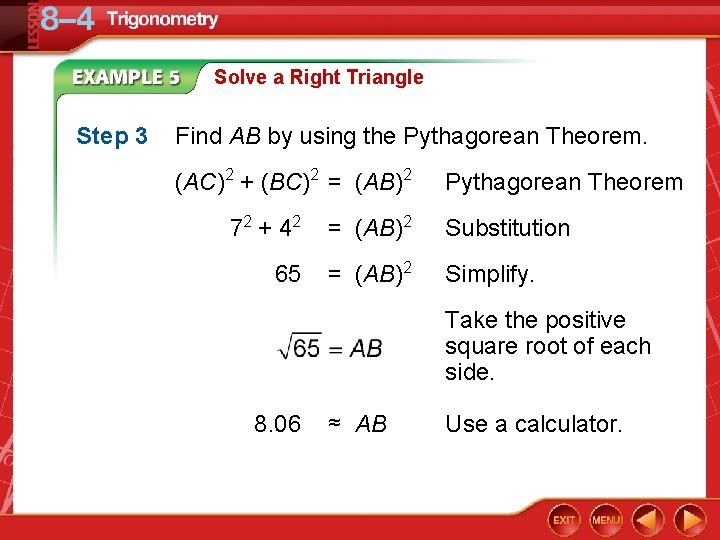Solve a Right Triangle Step 3 Find AB by using the Pythagorean Theorem. (AC)2 + (BC)2 = (AB)2 Pythagorean Theorem 72 + 4 2 = (AB)2 Substitution 65 = (AB)2 Simplify. Take the positive square root of each side. 8. 06 ≈ AB Use a calculator.Solve a Right Triangle So, the measure of AB is about 8. 06. Answer: m A ≈ 30, m B ≈ 60, AB ≈ 8. 06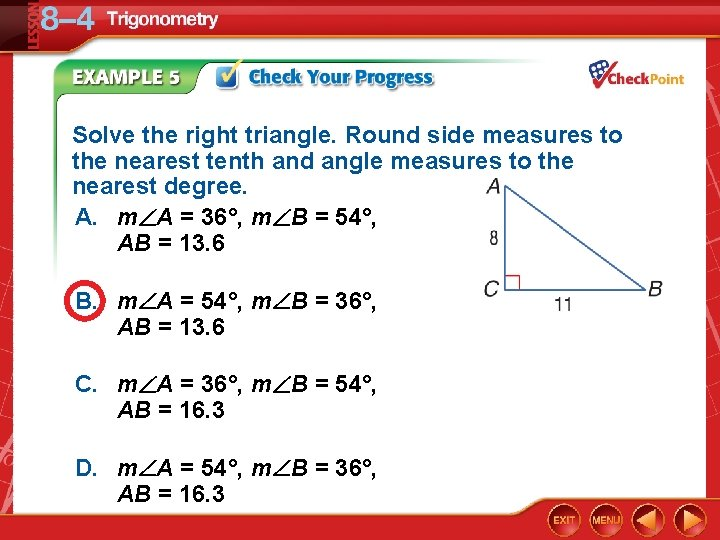Solve the right triangle. Round side measures to the nearest tenth and angle measures to the nearest degree. A. m A = 36°, m B = 54°, AB = 13. 6 B. m A = 54°, m B = 36°, AB = 13. 6 C. m A = 36°, m B = 54°, AB = 16. 3 D. m A = 54°, m B = 36°, AB = 16. 3LESSON 8– 4 Trigonometry